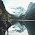## Rosheena's rate and unit rate problem

Math Homework

Group Problem:

If the are 6 girls per 2 boys, how much girls would there be if there if there is just one boy?

Own Problem:
Rate:
If there 9 periods per day, how much is the total period in 30 days?

2). If Isabelle worked for 8 hours per day, what is the total hours she earned, if she had work 28 days straight?

Unit Rate:
If every car in the block have 5 passenger each, what is the total amount of passenger if there are 15 cars on the block?

2). If Jerry have 27 papers and 9 pencils how much pencil does each paper get?

5:00 AM

#### 5 comments :

1.there are 3 girls per 1 boy 6/2=3

2.If the are 20 girls per 4 boys, how much girls would there be if there if there is just 2 boy?

1.10 girls because 20 divided by 2 = 10
thanks aisley

3.Here's the question for no.2:
~Each pencil will have 3 papers because 27/9 is equal to 3 so that means there will be 3 papers.

Here's a question:
If every car in the block have 4 passenger each, what is the total amount of passenger if there are 24 cars on the block?

1.there are 96 passenger in 24 cars on the block because 24.4=96
thanks justine